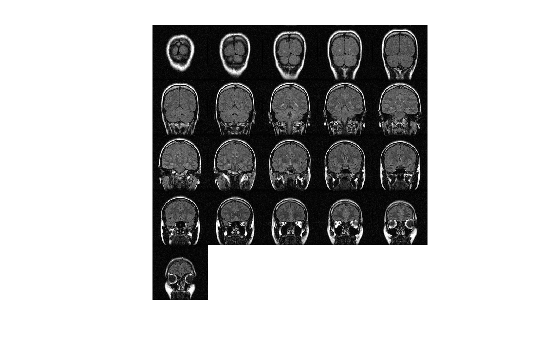# fspecial3

Create predefined 3-D filter

Since R2018b

## Syntax

``h = fspecial3(type)``
``h = fspecial3('average',hsize)``
``h = fspecial3('ellipsoid',semiaxes)``
``h = fspecial3('gaussian',hsize,sigma)``
``h = fspecial3('laplacian',gamma1,gamma2)``
``h = fspecial3('log',hsize,sigma)``
``h = fspecial3('prewitt',direction)``
``h = fspecial3('sobel',direction)``

## Description

````h = fspecial3(type)` creates a three-dimensional filter `h` of the specified `type`. Some of the filter types have optional additional parameters, shown in the following syntaxes. `fspecial3` returns `h` as a correlation kernel, which is the appropriate form to use with `imfilter`.```
````h = fspecial3('average',hsize)` returns an averaging filter `h` of size `hsize`. Not recommended. Use `imboxfilt3` instead.```

example

````h = fspecial3('ellipsoid',semiaxes)` returns an ellipsoidal averaging filter with the length of the principal semiaxes specified by `semiaxes`. The filter `h` is returned in an array of size `2*ceil(semiaxes)+1`.```
````h = fspecial3('gaussian',hsize,sigma)` returns a Gaussian lowpass filter of size `hsize` with standard deviation `sigma`. Not recommended. Use `imgaussfilt3` instead.```
````h = fspecial3('laplacian',gamma1,gamma2)` returns a 3-by-3-by-3 filter approximating the shape of the three-dimensional Laplacian operator. `gamma1` and `gamma2` control the shape of the Laplacian .```
````h = fspecial3('log',hsize,sigma)` returns a Laplacian of Gaussian filter of size `hsize` with standard deviation `sigma`. ```
````h = fspecial3('prewitt',direction)` returns a 3-by-3-by-3 filter that emphasizes gradients in the specified direction.```

example

````h = fspecial3('sobel',direction)` returns a 3-by-3-by-3 filter that emphasizes gradients in the specified direction and smooths the other directions .```

## Examples

collapse all

Load a 3-D grayscale MRI volume. Display the planes of the volume.

```load mristack; montage(mristack,'BackgroundColor','w')```Create a 3-D ellipsoidal filter. Specify a semiaxis length of 7 pixels in the y (rows) and x (columns) directions, and a semiaxis length of 3 pixels in the z (planes) direction.

`H = fspecial3('ellipsoid',[7 7 3]);`

Smooth the volume with the filter.

`volSmooth = imfilter(mristack,H,'replicate');`

Display the planes of the smoothed volume.

`montage(volSmooth,'BackgroundColor','w')`Load an MRI volume. This volume is stored as a 4-D array with a singleton dimension. Create a 3-D grayscale volume by using the `squeeze` function to remove the singleton dimension.

```load mri; V = squeeze(D);```

Display the planes of the volume.

`montage(D,'BackgroundColor','w')`Create a 3-D Sobel filter that detects horizontal edges in the volume. Horizontal edges appear where there is a large gradient magnitude in the y direction, so specify the direction of the Sobel filter as `'Y'`. The Sobel filter smooths the gradient in the x and z directions.

`H = fspecial3('sobel','Y');`

Filter the volume with the 3-D Sobel filter.

`edgesHor = imfilter(V,H,'replicate');`

Display the planes of the filtered volume.

`montage(edgesHor)`## Input Arguments

collapse all

Type of filter, specified as one of the following values:

Value

Description

`'average'`

Averaging filter. Not recommended. Use `imboxfilt3` instead.

`'ellipsoid'`

Ellipsoidal averaging filter

`'gaussian'`

Gaussian lowpass filter. Not recommended. Use `imgaussfilt3` instead.

`'laplacian'`

Approximates the three-dimensional Laplacian operator

`'log'`

Laplacian of Gaussian filter

`'prewitt'`

Prewitt edge-emphasizing filter

`'sobel'`

Sobel edge-emphasizing filter

Data Types: `char` | `string`

Size of the filter, specified as a positive integer or 3-element vector of positive integers. Use a vector to specify the number of rows, columns, and planes in `h`. Use a scalar to specify the side length of a cube.

For the `'gaussian'` and `'log'` filter types, if you specify `hsize` as `[]`, then `fspecial3` creates a filter with a default size of `2*ceil(2*sigma)+1`.

Data Types: `double`

Semiaxes length of an ellipsoidal filter, specified as a positive number or 3-element vector of positive numbers. Use a vector to specify the length of the three principal semiaxes in rows, columns, and planes. These values correspond to length in the Cartesian y, x, and z directions, respectively. Use a scalar to specify the radius of a sphere.

Data Types: `double`

Standard deviation of Gaussian filter, specified as a positive number or 3-element vector of positive numbers. If you specify a scalar, then `fspecial3` creates a cubic Gaussian kernel.

Data Types: `double`

Shape of the Laplacian, specified as a scalar in the range [0 1]. The sum of `gamma1` and `gamma2` must not exceed 1.

Data Types: `double`

Direction of gradients for Prewitt and Sobel filtering, specified as `'X'`, `'Y'`, or `'Z'`.

Data Types: `char` | `string`

## Output Arguments

collapse all

Correlation kernel, returned as a numeric array.

Data Types: `double`

 Lindeberg, T., Scale-Space Theory in Computer Vision. Boston, MA: Kluwer Academic Publishers, 1994.

 Geometry-Driven Diffusion in Computer Vision. Edited by B. M. ter Haar Romeny. Boston, MA: Kluwer Academic Publishers, 1994.

 Engel, K., M. Hadwiger, J. M. Kniss, C. Rezk-Salama, and D. Weiskopf. Real-Time Volume Graphics. Wellesley, MA: A K Peters, Ltd., 2006, pp. 112–114.